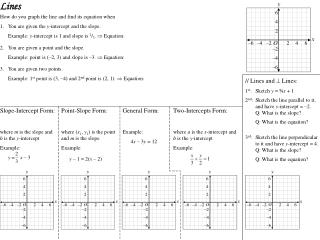DownloadDownload PresentationLines

# Lines

Download Presentation## Lines

- - - - - - - - - - - - - - - - - - - - - - - - - - - E N D - - - - - - - - - - - - - - - - - - - - - - - - - - -
##### Presentation Transcript

1. Lines y 6 4 2 x O –6 –4 –2 2 4 6 y y y y y –2 6 6 6 6 6 –4 4 4 4 4 4 –6 2 2 2 2 2 x x x x x O O O O O –6 –6 –6 –6 –6 –4 –4 –4 –4 –4 –2 –2 –2 –2 –2 2 2 2 2 2 4 4 4 4 4 6 6 6 6 6 –2 –2 –2 –2 –2 –4 –4 –4 –4 –4 –6 –6 –6 –6 –6 How do you graph the line and find its equation when 1. You are given the y-intercept and the slope. Example: y-intercept is 1 and slope is 2/3.  Equation: 2. You are given a point and the slope. Example: point is (–2, 3) and slope is –3.  Equation: 3. You are given two points. Example: 1st point is (3, –4) and 2nd point is (2, 1).  Equation: // Lines and  Lines: 1st: Sketch y = ¾x + 1 2nd: Sketch the line parallel to it, and have y-intercept = –2. Q: What is the slope? Q: What is the equation? 3rd: Sketch the line perpendicular to it and have y-intercept = 4. Q: What is the slope? Q: What is the equation? Slope-Intercept Form: where m is the slope and b is the y-intercept Example: y = x – 3 Point-Slope Form: where (x1, y1) is the point and m is the slope Example: y – 1 = 2(x – 2) General Form: Example: 4x – 3y = 12 Two-Intercepts Form: where a is the x-intercept and b is the y-intercept Example: